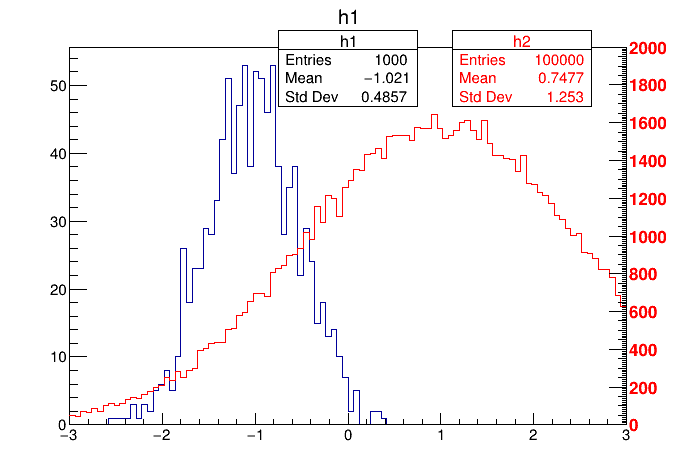Example of a canvas showing two histograms with different scales. The second histogram is drawn in a transparent pad

Author: Rene Brun
This notebook tutorial was automatically generated with ROOTBOOK-izer from the macro found in the ROOT repository on Thursday, May 19, 2022 at 08:19 AM.

In :
TCanvas *c1 = new TCanvas("c1","transparent pad",200,10,700,500);

TH1F *h1 = new TH1F("h1","h1",100,-3,3);
TH1F *h2 = new TH1F("h2","h2",100,-3,3);
TRandom r;
for (Int_t i=0;i<100000;i++) {
Double_t x1 = r.Gaus(-1,0.5);
Double_t x2 = r.Gaus(1,1.5);
if (i <1000) h1->Fill(x1);
h2->Fill(x2);
}
h1->Draw();
pad1->Update(); //this will force the generation of the "stats" box
TPaveStats *ps1 = (TPaveStats*)h1->GetListOfFunctions()->FindObject("stats");
ps1->SetX1NDC(0.4); ps1->SetX2NDC(0.6);
c1->cd();


Compute the pad range with suitable margins

In :
Double_t ymin = 0;
Double_t ymax = 2000;
Double_t dy = (ymax-ymin)/0.8; //10 per cent margins top and bottom
Double_t xmin = -3;
Double_t xmax = 3;
Double_t dx = (xmax-xmin)/0.8; //10 per cent margins left and right
h2->SetLineColor(kRed);
h2->Draw("][sames");
TPaveStats *ps2 = (TPaveStats*)h2->GetListOfFunctions()->FindObject("stats");
ps2->SetX1NDC(0.65); ps2->SetX2NDC(0.85);
ps2->SetTextColor(kRed);


Draw axis on the right side of the pad

In :
TGaxis *axis = new TGaxis(xmax,ymin,xmax,ymax,ymin,ymax,50510,"+L");
axis->SetLabelColor(kRed);
axis->Draw();


Draw all canvases

In :
gROOT->GetListOfCanvases()->Draw()